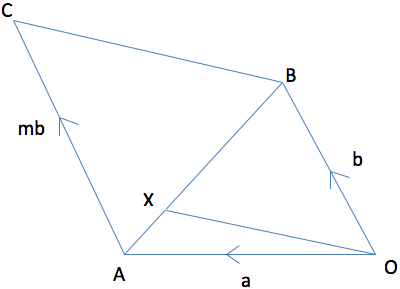SEARCH HOMEMath Central Quandaries & Querieshi...my name is Aishwaarya and i am a student from India.. My problem is in vector geometry which i am sending u my an attachment including a diagram.. Please Please help me.. Thanks AishwaryaHere is what Aishwarya sent usQuestion is-
AC is parallel to OB
Vector AX= 1/4 of vector AB
Vector OA= a
Vector Define a new point Y on BC so that XY is parallel to OB (and to AC). Then YXOB is a parallelogram, which means you know the length of XY. Then compare the similar triangles ABC and XBC -- the sides of ABC are m times as big as the sides of XBC.OB= b and
Vector AC= mb
Given that OX is parallel to BC, find the value of ‘m’

Aishwarya,

Define a new point Y on BC so that XY is parallel to OB (and to AC). Then YXOB is a parallelogram, which means you know the length of XY. Then compare the similar triangles ABC and XBC -- the sides of ABC are m times as big as the sides of XBC.

ChrisMath Central is supported by the University of Regina and The Pacific Institute for the Mathematical Sciences.